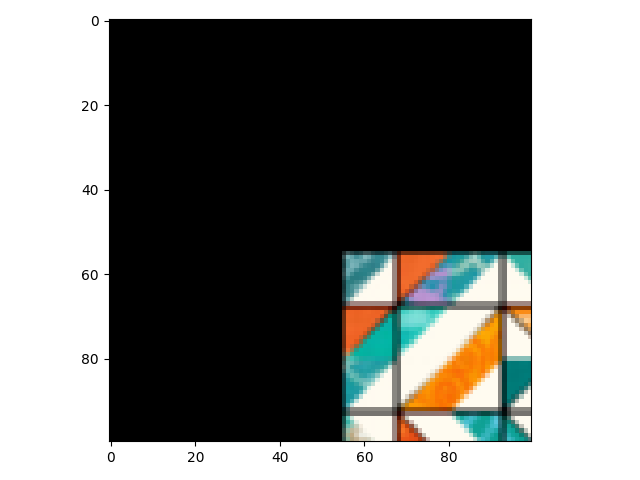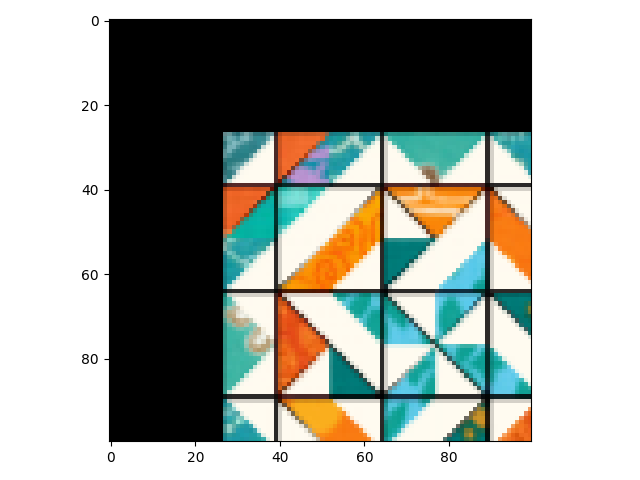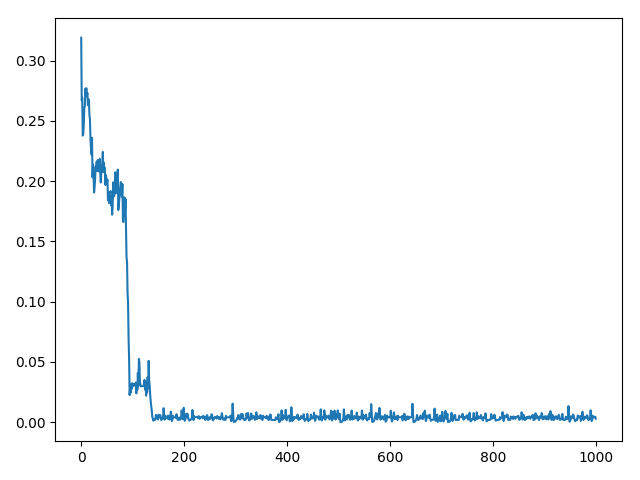# Learning Translation with Kornia

Hi,
I have been trying to learn translation (x, y) parameters with Kornia in the following manner:

``````class DTranslation(nn.Module):
def __init__(self, x_translation, y_translation):
super(DTranslation, self).__init__()
self.translations = torch.stack([x_translation, y_translation], 1)
self.angle = torch.tensor()

def forward(self, input):
_, _, h, w = input.shape
if self.angle.shape != input.shape:
angle = self.angle.repeat(input.shape)
else:
angle = self.angle

if self.translations.shape != input.shape:
translations = self.translations.repeat([input.shape, 1])
else:
translations = self.translations

translations[:, 0] *= h
translations[:, 1] *= w

# define the rotation center
center = torch.ones(2)
center[..., 0] = input.shape / 2  # x
center[..., 1] = input.shape / 2  # y
center = center.repeat(input.shape, 1)

# define the scale factor
scale = torch.ones(input.shape)

# compute the transformation matrix
M = kornia.get_rotation_matrix2d(center, -angle, scale)

# Translate
M[..., 2] += translations  # tx/ty

# apply the transformation to original image
out = kornia.warp_affine(input, M, dsize=(h, w))

return out

tx = torch.tensor([0.3], dtype=torch.float32)

ty = torch.tensor([-0.2], dtype=torch.float32)

translation = DTranslation(x_translation=tx_p, y_translation=ty_p)

criterion = nn.MSELoss()

loss = criterion(x, translation(x))
loss.backward()
optimizer.step()
``````

The first backward call passes, but the second one fails:

``````RuntimeError: Trying to backward through the graph a second time, but the saved intermediate results have already been freed. Specify retain_graph=True when calling backward the first time.
``````

`````` loss.backward(retain_graph=True)
``````

``````RuntimeError: one of the variables needed for gradient computation has been modified by an inplace operation: [torch.FloatTensor ] is at version 1; expected version 0 instead. Hint: enable anomaly detection to find the operation that failed to compute its gradient, with torch.autograd.set_detect_anomaly(True).
``````

I also tried to avoid the inplace operation:

``````        M[..., 2] += translations  # tx/ty
``````

``````shape = list(M.shape)
shape[-1] -= 1
M = M + torch.cat([torch.zeros(shape), translations.unsqueeze(-1)], 2)
``````

but got the same errors.

Maybe another inplace operation causes this ? e.g. https://github.com/kornia/kornia/blob/5a736409a9a133da27c3dfa581bba2bc71f27286/kornia/geometry/conversions.py#L122

Or is it something else ?

It is worth mentioning that I do manage to backpropagated through rotation angle and shear (x, y) but only translation seems to be the problem, i.e. when I comment out

``````        M[..., 2] += translations  # tx/ty
``````

No errors occur, but of course I cannot learn the translation parameters either.

Any thoughts ?

My guess is that defining the constant outside nn.Module breaks the graph. Once you define them inside nn.Module they are gonna be registered as buffers and it sounds bad to me.

Why don’t you just define them as regular nn.Parameters and pass the nn.Module to the optimizer?
Anyway, can you provide a runnable script?

WRT the inplace ops, indeed that M[…,2] is not allowed.

Thank you.

Please note that I manage to learn rotation angle while the parameters are passed to the nn.Module so this doesn’t seem to be the problem.

Attached is a runbable code (originally a notebook):

``````import kornia; print(kornia.__version__)

# In:

import torch
import torchvision
import torchvision.transforms as transforms

transform = transforms.Compose(
[transforms.ToTensor(),
transforms.Normalize((0.5, 0.5, 0.5), (0.5, 0.5, 0.5))])

bs= 4#096

classes = ('plane', 'car', 'bird', 'cat',
'deer', 'dog', 'frog', 'horse', 'ship', 'truck')

# In:

from torch import nn
class DRotation(nn.Module):
def __init__(self, angle):
super(DRotation, self).__init__()
self.angle = angle

def forward(self, input):
if self.angle.shape != input.shape:
angle = self.angle.repeat(input.shape)
else:
angle = self.angle

# define the rotation center
center = torch.ones(2)
center[..., 0] = input.shape / 2  # x
center[..., 1] = input.shape / 2  # y
center = center.repeat(input.shape, 1)

# define the scale factor
scale = torch.ones(input.shape)

# compute the transformation matrix
M = kornia.get_rotation_matrix2d(center, -angle, scale)

# apply the transformation to original image
_, _, h, w = input.shape
out = kornia.warp_affine(input, M, dsize=(h, w))

return out

# In:

class DTranslation(nn.Module):
def __init__(self, x_translation, y_translation):
super(DTranslation, self).__init__()
self.translations = torch.stack([x_translation, y_translation], 1)
self.angle = torch.tensor()

def forward(self, input):
_, _, h, w = input.shape
if self.angle.shape != input.shape:
angle = self.angle.repeat(input.shape)
else:
angle = self.angle

if self.translations.shape != input.shape:
translations = self.translations.repeat([input.shape, 1])
else:
translations = self.translations

translations[:, 0] *= h
translations[:, 1] *= w

# define the rotation center
center = torch.ones(2)
center[..., 0] = input.shape / 2  # x
center[..., 1] = input.shape / 2  # y
center = center.repeat(input.shape, 1)

# define the scale factor
scale = torch.ones(input.shape)

# compute the transformation matrix
M = kornia.get_rotation_matrix2d(center, -angle, scale)

# Translate
M[..., 2] += translations  # tx/ty
#         shape = list(M.shape)
#         shape[-1] -= 1
#         M = M + torch.cat([torch.zeros(shape), translations.unsqueeze(-1)], 2)

# apply the transformation to original image
out = kornia.warp_affine(input, M, dsize=(h, w))

return out

# In:

from torch.nn.parameter import Parameter

class LearnableAug(nn.Module):
def __init__(self, aug_names=[]):
super(LearnableAug, self).__init__()

self.fixed_transforms = []
self.learnable_transforms = []
self.have_entropy = []
self._param_groups = []
self.lrs = []
self.bounds= {}
self.eps = 0 #1e-6

if 'translation' in aug_names:
self.register_buffer('_x_translation', var)
self.register_buffer('_y_translation', var)

self.fixed_transforms.append(DTranslation(
x_translation=self._buffers['_x_translation'],
y_translation=self._buffers['_y_translation'],
))

var = torch.tensor(, dtype=torch.float32)
self.register_parameter(name='x_translation', param=param)

var = torch.tensor(, dtype=torch.float32)
self.register_parameter(name='y_translation', param=param)

self.learnable_transforms.append(DTranslation(
x_translation=self._parameters['x_translation'],
y_translation=self._parameters['y_translation'],
))

self._param_groups.append(['x_translation'])
self.lrs.append(1)
self.bounds['x_translation'] = (-0.5, 0.5)

self._param_groups.append(['y_translation'])
self.lrs.append(1)
self.bounds['y_translation'] = (-0.5, 0.5)

if 'rotation' in aug_names and 'fixed_affine' not in aug_names:
# Fixed Rotation
self.register_buffer('_angle', var)
self.fixed_transforms.append(DRotation(var))

var = torch.tensor(, dtype=torch.float32)
self.register_parameter(name='angle', param=param)
self.learnable_transforms.append(DRotation(param))
self._param_groups.append(['angle'])
self.lrs.append(1)
self.bounds['angle'] = (-60, 60)

self.fixed_transforms = torch.nn.Sequential(*self.fixed_transforms)
self.learnable_transforms = torch.nn.Sequential(*self.learnable_transforms)

def apply_constraints(self):
for param_name, bounds in self.bounds.items():
if self._parameters[param_name] < bounds:
if isinstance(bounds, torch.Tensor):
self._parameters[param_name].data = bounds.data
else:
bound_tensor = torch.ones_like(self._parameters[param_name]) * bounds
self._parameters[param_name].data = bound_tensor.data

if self._parameters[param_name] > bounds:
if isinstance(bounds, torch.Tensor):
self._parameters[param_name].data = bounds.data
else:
bound_tensor = torch.ones_like(self._parameters[param_name]) * bounds
self._parameters[param_name].data = bound_tensor.data

def forward(self, x, fixed=False):
if not fixed:
self.apply_constraints()
out = self.learnable_transforms(x)
else:
out = self.fixed_transforms(x)
return out

@property
def param_groups(self):
return self._param_groups

def get_params_for_optimizer(self):
param_groups = []
for param_group, lr in zip(self._param_groups, self.lrs):
param_groups.append(dict(params=[self._parameters[name] for name in param_group], lr=lr))

return param_groups

# In:

from IPython.display import clear_output

from time import sleep
from torch import optim
device = torch.device('cuda')

augs = LearnableAug(['rotation'])
# augs = LearnableAug(['translation'])
criterion = nn.MSELoss()
scheduler = optim.lr_scheduler.CosineAnnealingWarmRestarts(optimizer, T_0=10)#, T_mult)

epochs = 1

for epoch in range(epochs):
images, labels = data

# perform the transforms
ft_images = augs(images, fixed=True)
dt_images = augs(images)

loss = criterion(ft_images, dt_images)
print(loss)
loss.backward()

optimizer.step()
scheduler.step((epoch * iters + i) / (epochs * iters))
``````

Soo I did a minimal example:

``````from torch import nn
import torch
import kornia
import imageio

import cv2
import matplotlib.pyplot as plt
import numpy as np

ex = cv2.resize(ex, (100, 100)).astype(np.float32)

torch.manual_seed(666)

def show(img):
if isinstance(img, np.ndarray):
pass
else:
img = img.permute(1, 2, 0).detach().cpu().numpy()
plt.imshow(img)
plt.show()

show(ex)

class DTranslation(nn.Module):
def __init__(self, x_translation, y_translation):
super(DTranslation, self).__init__()
self.translations = nn.Parameter(torch.stack([x_translation, y_translation], 1))
self.angle = torch.tensor()

def forward(self, input, train=True):
_, _, h, w = input.shape
if self.angle.shape != input.shape:
angle = self.angle.repeat(input.shape)
else:
angle = self.angle

if self.translations.shape != input.shape:
translations = self.translations.repeat([input.shape, 1])
else:
translations = self.translations
if train:
translations = torch.sigmoid(translations) * torch.Tensor([h, w])
else:
translations = translations * torch.Tensor([h, w])
# define the rotation center
center = torch.ones(2)
center[..., 0] = input.shape / 2  # x
center[..., 1] = input.shape / 2  # y
center = center.repeat(input.shape, 1)

# define the scale factor
scale = torch.ones(input.shape)

# compute the transformation matrix
M = kornia.get_rotation_matrix2d(center, -angle, scale)

# Translate
shape = list(M.shape)
shape[-1] -= 1
M = M + torch.cat([torch.zeros(shape), translations.unsqueeze(-1)], 2)

# apply the transformation to original image
out = kornia.warp_affine(input, M, dsize=(h, w), padding_mode='zeros')

return out

tx = torch.tensor([-1], dtype=torch.float32)

ty = torch.tensor([-1], dtype=torch.float32)

translation = DTranslation(x_translation=tx, y_translation=ty)

class Corr(nn.Module):
def forward(self, x, pred):
x = x.flatten()
p = pred.flatten()
x_n = torch.norm(x)
p_n = torch.norm(p)

criterion = nn.L1Loss()
# criterion = nn.CosineSimilarity(1)
img = torch.from_numpy(ex).permute(2, 0, 1)[None, ...]
inst = DTranslation(torch.tensor([0.2], dtype=torch.float32),
torch.tensor([0.2], dtype=torch.float32))

gt = inst(img)
show(gt)
for i in range(1000):
result = translation(img, train=True)
if i % 10 == 0:
show(result)
loss = criterion(gt.view(1, -1), result.view(1, -1))

loss.backward()
f'Loss: {loss.item()}, '
f'Value: {torch.sigmoid(translation.translations.data)}')
optimizer.step()
``````

But i have to say i don’t figure out why it doesn’t optimize it.
Gradients are not None, thus it properly prop.
The rot matrix is ok
I’ve tried to maximize corr instead of minimize euclidian but yet the same

I’ll have another look later

Thank you. I wish I knew the answer. To be honest I asked the same question in Kornia’s Github repo and shared the code there:

I hope they find out the reason and correct this.

I think it’s more theoretical than any other thing.
If you run the example i wrote you will see it kinda get stuck in a local minima.

Thank you for your code. I ran it. Indeed it doesn’t raise that error, yet it doesn’t train.
Actually, Instead of taking all the changes you suggested, I took my code and changed only the line:

``````self.translations = torch.stack([x_translation, y_translation], 1)
``````

To:

``````self.translations = nn.Parameter(torch.stack([x_translation, y_translation], 1))
``````

and indeed the error went away. Yet the gradients w.r.t the original translation tensors remain zero.
I wonder if the constructor nn.Parameter() somehow duplicates the tensor such that the original one is detached from the graph hence no error occur yet neither gradients are back-propagated properly.

I mean, it should be working also without this change, as done in the rotation example in my code.

Well,
I don’t remember the details of Parameter but my guess is it detach.
It’s not pythonic->“pytorchnic” to define a parameter outside me module. If that is the case, then you don’t really need to wrap everything in a nn.Module. You can just use a standard function.

When you assign a torch tensor to a nn.Module, it internally calls register buffer. If torch is promoting the stack of to parameters into a tensor, the resulting one is being assigned as a buffer.

Anyway the input is gonna be a leaf node. If you run a gradient anomaly detector it will rise an error since gradients doesn’t flow to the input due to inplace ops.
Howver you are only using inplace ops to build the rotation matrix. The ops related to the translation vector are not affected and that’s why gradients flow down there.

Besides, the rotation matrix is ok. Ones in the diag + the translation vector .

My point is that:
GT:1st prefDpending of the learning rate it jumps around that point
or diverge

With a bit more of analysis.

``````from torch import nn
import torch
import kornia
import imageio

import cv2
import matplotlib.pyplot as plt
import numpy as np

ex = cv2.resize(ex, (100, 100)).astype(np.float32)

torch.manual_seed(666)

def show(img):
if isinstance(img, np.ndarray):
pass
else:
img = img.permute(1, 2, 0).detach().cpu().numpy()
plt.imshow(img)
plt.show()

show(ex)

class DTranslation(nn.Module):
def __init__(self, x_translation, y_translation):
super(DTranslation, self).__init__()
self.translations = nn.Parameter(torch.stack([x_translation, y_translation], 1))
self.angle = torch.tensor()

def forward(self, input, train=True):
_, _, h, w = input.shape
if self.angle.shape != input.shape:
angle = self.angle.repeat(input.shape)
else:
angle = self.angle

if self.translations.shape != input.shape:
translations = self.translations.repeat([input.shape, 1])
else:
translations = self.translations
if train:
translations = torch.sigmoid(translations) * torch.Tensor([h, w])
else:
translations = translations * torch.Tensor([h, w])
# define the rotation center
center = torch.ones(2)
center[..., 0] = input.shape / 2  # x
center[..., 1] = input.shape / 2  # y
center = center.repeat(input.shape, 1)

# define the scale factor
scale = torch.ones(input.shape)

# compute the transformation matrix
M = kornia.get_rotation_matrix2d(center, -angle, scale)

# Translate
shape = list(M.shape)
shape[-1] -= 1
M = M + torch.cat([torch.zeros(shape), translations.unsqueeze(-1)], 2)
# apply the transformation to original image
out = kornia.warp_affine(input, M, dsize=(h, w), padding_mode='zeros')

return out

tx = torch.tensor([-1], dtype=torch.float32)

ty = torch.tensor([-1], dtype=torch.float32)

translation = DTranslation(x_translation=tx, y_translation=ty)

class Corr(nn.Module):
def forward(self, x, pred):
x = x.flatten()
p = pred.flatten()
x_n = torch.norm(x)
p_n = torch.norm(p)

criterion = nn.MSELoss()
# criterion = nn.CosineSimilarity(1)
optimizer = torch.optim.SGD(translation.parameters(), lr=0.75)
img = torch.from_numpy(ex).permute(2, 0, 1)[None, ...]
inst = DTranslation(torch.tensor([0.2], dtype=torch.float32),
torch.tensor([0.2], dtype=torch.float32))

gt = inst(img)
show(gt)
loss_h = []
for i in range(100):
result = translation(img, train=True)
if i % 10 == 0:
show(result)
loss = criterion(gt.view(1, -1), result.view(1, -1))
loss_h.append(loss.item())
loss.backward()
f'Loss: {loss.item()}, '
f'Value: {torch.sigmoid(translation.translations.data)}')
optimizer.step()
plt.plot(loss_h)
plt.show()
``````

I just went forward SGD to avoid statistical optimizers.It get stuck.
GTPred NYou can try to reduce LR through time, using an scheduler or manually.
It’s somehow ill posed
For lr=0.5It reaches a good result but diverge in the end.
Maybe an iterative scheme which grabs the best result can help u

Great !

Choosing lr=0.1 does the trick:

Still I fail to understand what the problem is. After all I determined the parameter in a nn.Module (LearnableAugs) and then passed it to be processed in an internal nn.Module that doesn’t have those registered as parameters. Nevertheless, I expect the gradients to flow properly as happening for all the other transforms I learn (e.g. rotation, shear, etc.). It’s a mystery to me.

I think you should predict directly the magnitude value.
Predicting a number between 0-1 makes gradients to be multiplied by 100.

It smoothes the curve.
The best result i got is the following one:
Which goes from the original position to (20,20)
But it’s really dependent on the LR.
LR 50 makes it to diverge and LR 10 makes it to stuck in a minima.``````from torch import nn
import torch
import kornia
import imageio

import cv2
import matplotlib.pyplot as plt
import numpy as np

ex = cv2.resize(ex, (100, 100)).astype(np.float32)

torch.manual_seed(666)

def show(img):
if isinstance(img, np.ndarray):
pass
else:
img = img.permute(1, 2, 0).detach().cpu().numpy()
plt.imshow(img)
plt.show()

show(ex)

class DTranslation(nn.Module):
def __init__(self, x_translation, y_translation):
super(DTranslation, self).__init__()
self.translations = nn.Parameter(torch.stack([x_translation, y_translation], 1))
self.angle = torch.tensor()

def forward(self, input, train=True):
_, _, h, w = input.shape
if self.angle.shape != input.shape:
angle = self.angle.repeat(input.shape)
else:
angle = self.angle

if self.translations.shape != input.shape:
translations = self.translations.repeat([input.shape, 1])
else:
translations = self.translations
if train:
# translations = translations * torch.Tensor([h, w])
translations = translations
else:
# translations = translations * torch.Tensor([h, w])
translations = translations
# define the rotation center
center = torch.ones(2)
center[..., 0] = input.shape / 2  # x
center[..., 1] = input.shape / 2  # y
center = center.repeat(input.shape, 1)

# define the scale factor
scale = torch.ones(input.shape)

# compute the transformation matrix
M = kornia.get_rotation_matrix2d(center, -angle, scale)

# Translate
shape = list(M.shape)
shape[-1] -= 1
M = M + torch.cat([torch.zeros(shape), translations.unsqueeze(-1)], 2)
# apply the transformation to original image
out = kornia.warp_affine(input, M, dsize=(h, w), padding_mode='zeros')

return out

tx = torch.tensor([1.], dtype=torch.float32)

ty = torch.tensor([1.], dtype=torch.float32)

translation = DTranslation(x_translation=tx, y_translation=ty)

class Corr(nn.Module):
def forward(self, x, pred):
x = x.flatten()
p = pred.flatten()
x_n = torch.norm(x)
p_n = torch.norm(p)

criterion = nn.MSELoss()
# criterion = nn.CosineSimilarity(1)
optimizer = torch.optim.SGD(translation.parameters(), lr=25)
img = torch.from_numpy(ex).permute(2, 0, 1)[None, ...]
inst = DTranslation(torch.tensor([20.], dtype=torch.float32),
torch.tensor([20.], dtype=torch.float32))

gt = inst(img)
show(gt)
loss_h = []
N=1000
for i in range(N):
result = translation(img, train=True)
if i % (N//100) == 0:
show(result)
loss = criterion(gt.view(1, -1), result.view(1, -1))
loss_h.append(loss.item())
loss.backward()
f'Loss: {loss.item()}, '
f'Value: {translation.translations.data}')
optimizer.step()
plt.plot(loss_h)
plt.show()
``````

The issue is solved, with both the kind help from @JuanFMontesinos and at https://github.com/kornia/kornia/issues/682

The only problem was placing `translations = torch.stack([x_translation, y_translation], 1)` at the init () rather than in the forward().

FYI all the inplace operations do not interfere with the differentiation as those are all differentiable, i,e, both:
`translations[:, 0] *= h translations[:, 1] *= w`
And:
`M[..., 2] += translations`

Eventually the working code is:

``````class DTranslation(nn.Module):
def __init__(self, x_translation, y_translation):
super(DTranslation, self).__init__()
self.x_translation = x_translation
self.y_translation = y_translation
self.angle = torch.tensor()

def forward(self, input):
_, _, h, w = input.shape
if self.angle.shape != input.shape:
angle = self.angle.repeat(input.shape)
else:
angle = self.angle

translations = torch.stack([self.x_translation, self.y_translation], 1)
if translations.shape != input.shape:
translations = translations.repeat([input.shape, 1])
else:
translations = translations

translations[:, 0] *= h
translations[:, 1] *= w

# define the rotation center
center = torch.ones(2)
center[..., 0] = input.shape / 2  # x
center[..., 1] = input.shape / 2  # y
center = center.repeat(input.shape, 1)

# define the scale factor
scale = torch.ones(input.shape)

# compute the transformation matrix
M = kornia.get_rotation_matrix2d(center, -angle, scale)

# Translate
M[..., 2] += translations  # tx/ty

# apply the transformation to original image
out = kornia.warp_affine(input, M, dsize=(h, w))

return out
``````

Closing this issue.